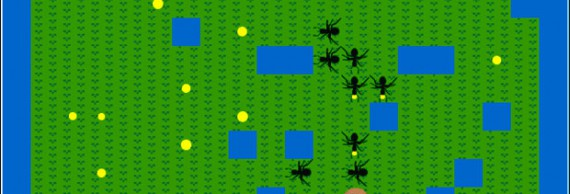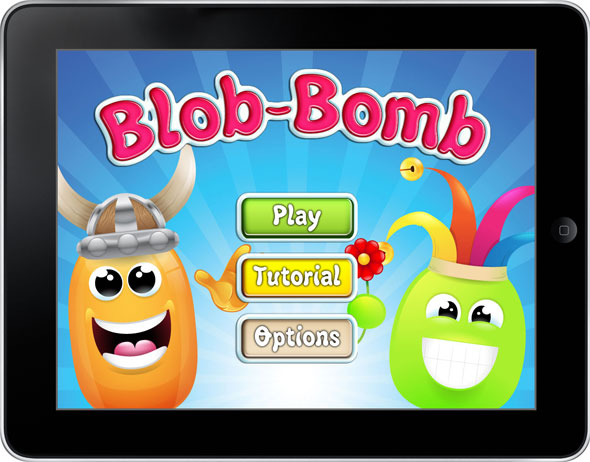# Addepar Ant AI & Blob-BombAddepar requests that internship applicants program an ant AI in Java. This was my first experience programming an AI, so I stuck with classic techniques like state machines and A* pathfinding to be sure I ended up with something functional. In this article, I’ll be documenting the challenge, my strategy, and possible improvements. I’ll also include a sneak-peek of my newest game project.

### The Rules

1. You start out with 3 ants on an anthill with 1 ant being generated every 10 turns.
2. Each turn, every ant can perform one of the following moves: Halt, Gather, Drop off, or move one square.
3. The ants can trade information if they are on the same square.
4. Ants can see one square in all four directions and the square they are standing on.
5. The goal is to collect ~80% of the food in as few turns as possible.

### My Strategy

1. Ants record all tile information they can, building up a mental map.
2. Ants trade and merge their mental maps whenever they land on the same tile.
3. Ants lean preferentially towards known food sources versus the unknown (distance breaks ties).
4. Ants determine the shortest route to a food source using the A* algorithm.

### Results

Overall, the AI functions pretty well – you can clearly see the ants are following a strategy. The limitations on what an ant can know about it’s environment made for a fun programming challenge.

If I had time to improve the AI, I’d probably look at optimizing how the ants balance known food sources versus discovery – This could be done by building a test harness that ran a couple days worth of tests and recorded the results depending on the “discovery constant.” This would allow a host of other optimizations by allowing me to test answering other questions like “Does sitting on the anthill for a certain number of ticks improve knowledge and in turn food acquisition?” This testing harness would probably provide the most bang-for-my-buck coding wise.

In the future, I’d like to try a bottom up approach to developing and see if that improves my ability to write more loosely coupled classes. I’m not terribly happy with the code organization. Looking into extending Java’s built-in data structures would probably improve code readability as well. Without further adieu, here is the code:

```123456789101112131415161718192021222324252627282930313233343536373839404142434445464748495051525354555657585960616263646566676869707172737475767778798081828384858687888990919293949596979899100101102103104105106107108109110111112113114115116117118119120121122123124125126127128129130131132133134135136137138139140141142143144145146147148149150151152153154155156157158159160161162163164165166167168169170171172173174175176177178179180181182183184185186187188189190191192193194195196197198199200201202203204205206207208209210211212213214215216217218219220221222223224225226227228229230231232233234235236237238239240241242243244245246247248249250251252253254255256257258259260261262263264265266267268269270271272273274275276277278279280281282283284285286287288289290291292293294295296297298299300301302303304305306307308309310311312313314315316317318319320321322323324325326327328329330331332333334335336337338339340341342343344345346347348349350351352353354355356357358359360361362363364365366367368369370371372373374375376377378379380381382383384385386387388389390391392393394395396397398399400401402403404405406407408409410411412413414415416417418419420421422423424425426427428429430431432433434435436437438439440441442443444445446447448449450451import ants.*;
import java.io.ByteArrayInputStream;
import java.io.ByteArrayOutputStream;
import java.io.DataInputStream;
import java.io.DataOutputStream;
import java.io.IOException;
import java.util.ArrayList;
import java.util.HashMap;
import java.util.HashSet;
import java.util.Iterator;
import java.util.Set;

public class MyAnt implements Ant{

private Brain MyBrain = new Brain();

public Action getAction(Surroundings surroundings){
// Always load up my brain with new information.
// Knowledge is Power!
Surroundings s = surroundings;
return MyBrain.getAction();
}

public byte[] send(){
// Sends a byte[] of a list of integers in the following order
// x, y, food, frontier? ... and so on
try {
return MyBrain.dataOutput();
} catch (IOException e) {
e.printStackTrace();
return null;
}
}

// Recieves a byte[] of a list of Integers in x, y, food, frontier? ... and so on order
// and sends it to the brain for processing.
try {
MyBrain.dataInput(data);
} catch (IOException e) {
e.printStackTrace();
}
}

// How a relative coordinate is stored in my HashMap
class Cell {
public Integer food;
public Integer x;
public Integer y;

public Integer f, g, h;
public Cell parent;

public Cell(Integer x, Integer y, Integer food) {
this.food = food;
this.x = x;
this.y = y;

this.f = 0;
this.g = 0;
this.h = 0;
this.parent = null;
}

public int hashCode() {
String s = x + ",,," + y;
return s.hashCode();
}

public String key() {
String k = x + "," + y;
return k;
}
}

class Map {
String antHill;
HashSet food;
HashSet frontier;
HashMap mapIndex;
Integer x,y;

public Map() {
this.x = 0;
this.y = 0;
this.antHill = xyToS(0, 0);
this.mapIndex = new HashMap();
this.mapIndex.put(antHill, new Cell(0, 0, 0));
this.food = new HashSet();
this.frontier = new HashSet();
}

// Gives a key for the current coordinates, offset by supplied xOff and yOff
// Offset of 0,0 would give the current coordinate's key
private String xyToS(Integer xOff, Integer yOff) {
return ((x+xOff) + "," + (y+yOff));
}

// Updates or creates a cell in our Ant's HashMap
private void updateSurrounding(String key, Tile t, Integer xx, Integer yy) {
if (mapIndex.get(key) != null) {
// Update the HashMap's cell
Cell c = mapIndex.remove(key);
c.food = t.getAmountOfFood();
if (c.food &lt;= 0)
food.remove(key);
mapIndex.put(key, c);
} else {
// Create a new cell for the hashmap
if (t.isTravelable()) {
Cell c = new Cell(xx, yy, t.getAmountOfFood());
mapIndex.put(key, c);
if (c.food &gt; 0)
}
}
}

// Takes as input all NEWS tiles and then adds them to your ant's internal map
public void setSurroundings(Tile N, Tile E, Tile W, Tile S) {
}

// Takes as input the current cell's food count and updates the ant's map
public void setCurrent(Integer food) {
Cell c = getCurrent();
if (food &lt;= 0)
this.food.remove(c);
c.food = food;
}

// Returns true if we are standing on food
public Boolean foodHere() {
return mapIndex.get(xyToS(0, 0)).food &gt; 0;
}

// Returns true if we are standing on the anthill
public Boolean homeHere() {
return antHill.equals(xyToS(0, 0));
}

// Gets the nearest cell from a HashSet of cells
private Cell closestTarget(HashSet m) {
Cell best = null;
Integer distance = Integer.MAX_VALUE;

if (!m.isEmpty()) {
Cell cur = getCurrent();
for (String key : m) {
if (estimateDistance(cur, mapIndex.get(key)) &lt; distance) {
best = mapIndex.get(key);
distance = estimateDistance(cur, best);
}
}
}
return best;
}

// Returns the cell our ant should move towards.
// Goes to the closest food source, unless an undiscovered cell is less than half
// the distance.
public Cell getBestTarget() {
// Find closest food and frontier cells
Cell bestFoodCell = closestTarget(food);
Cell bestFrontierCell = closestTarget(frontier);

if (estimateDistance(getCurrent(), bestFrontierCell)*2 &lt;= estimateDistance(getCurrent(), bestFoodCell))
return bestFrontierCell;
else
return bestFoodCell;
}

// Returns the current Cell we are standing on
public Cell getCurrent() {
return mapIndex.get(xyToS(0, 0));
}

// Returns the anthill cell
public Cell getHome() {
return mapIndex.get(antHill);
}

// Based on a neighboring coordinate destination, this returns which direction we need
// to move to get there.
public Direction move(String key) {
String[] k = key.split(",");
Integer kx = Integer.parseInt(k);
Integer ky = Integer.parseInt(k);
frontier.remove(key);
if (y+1 == ky) {
this.y += 1;
return Direction.NORTH;
}
else if (x+1 == kx) {
this.x += 1;
return Direction.EAST;
}
else if (x-1 == kx) {
this.x -= 1;
return Direction.WEST;
}
else {
this.y -= 1;
return Direction.SOUTH;
}
}

// Returns an ArrayList of all known neighboring cells
public ArrayList getNeighbors(String key) {
String[] k = key.split(",");
Integer x = Integer.parseInt(k);
Integer y = Integer.parseInt(k);
ArrayList ret = new ArrayList();
if (mapIndex.get(x+","+(y+1)) != null)
if (mapIndex.get((x+1)+","+y) != null)
if (mapIndex.get((x-1)+","+y) != null)
if (mapIndex.get(x+","+(y-1)) != null)

return ret;
}

public Integer estimateDistance(Cell cell1, Cell cell2) {
if (cell1 == null || cell2 == null)
return Integer.MAX_VALUE;
return Math.abs(cell1.x - cell2.x) + Math.abs(cell1.y - cell2.y);
}

// Updates a cell's food if it's less than what is already in our map
private void updateCell(String key, Integer food) {
Cell c = mapIndex.get(key);
c.food = Math.min(food, c.food);
mapIndex.put(key, c);
}

// Merge list of Integers into our ant's map
// List: x, y, food, frontier? ... and so on
while(itr.hasNext()) {
Integer x = itr.next();
Integer y = itr.next();
Integer food = itr.next();
Boolean frontier = (1 == itr.next());
String k = x+","+y;

// If we already have the cell, update it.
// Otherwise add a new cell and put it in the appropriate frontier or food list
if(mapIndex.containsKey(k)) {
updateCell(k, food);
if (food &lt;= 0)
this.food.remove(k);
} else {
mapIndex.put(k, new Cell(x, y, food));
if (food &gt; 0)

if (frontier)
}

}
}
}

enum STATE { Exploring, GoingHome }

class Brain {
Map map;
STATE state;

public Brain() {
map = new Map();
state = STATE.Exploring;
}

// Takes a byte[] that's composed of an ArrayList and
// inputs it into our ant's brain.
// Integer Format: x, y, food, frontier? ... and so on
public void dataInput(byte[] in) throws IOException {
ByteArrayInputStream bais = new ByteArrayInputStream(in);
DataInputStream inp = new DataInputStream(bais);
while (inp.available() &gt; 0) {
}
}

// Outputs an ArrayList as a byte[] to send
// to another ant.
// Format: x, y, food, frontier? ... and so on
public byte[] dataOutput() throws IOException {
for (Cell c : map.mapIndex.values()) {
if(map.frontier.contains(c.key()))
else
}
// write to byte array
ByteArrayOutputStream baos = new ByteArrayOutputStream();
DataOutputStream out = new DataOutputStream(baos);
for (Integer element : quads) {
out.writeUTF(element.toString());
}
byte[] bytes = baos.toByteArray();
return bytes;
}

// Load the current tile's data into our brain
map.setCurrent(food);
}

// Load our neighboring tile's data into our brain
public void loadSurroundings(Tile N, Tile E, Tile W, Tile S) {
map.setSurroundings(N, E, W, S);
}

// Main Strategy Center
// 2 States - Exploration and GoingHome
// If an ant is GoingHome, it means the ant has collected food to drop off.
// After dropping off, the ant's state is set to Exploring.
// When exploring, the ant moves towards known food sources or undiscovered areas
// (going towards food if its less than twice as far as the nearest undiscovered area).
// If it finds food on the way, it picks it up and head's home otherwise it gets to its destination,
// picking up food if it's still there or else it finds a new best destination.
//
// Routes are created with the A* algorithm
public Action getAction() {
if (state == STATE.GoingHome) {
if (route.isEmpty() &amp;&amp; !map.homeHere())
route = aStar(map.getCurrent(), map.getHome());

// 1. Drop off food if we're home
// 2. Otherwise continue heading home
if (map.homeHere()) {
state = STATE.Exploring;
return Action.DROP_OFF;
} else {
return Action.move(map.move(route.removeLast()));
}
}
else if (state == STATE.Exploring)
{
// 1. Pickup food if possible
// 2. Explore with food target taking precedence over exploring
if (map.foodHere() &amp;&amp; !map.homeHere()) {
// Pick up, set state to picking up (save prev val)
route.clear();
state = STATE.GoingHome;
return Action.GATHER;
} else {
// frontier/food explore
if (route.isEmpty())
route = aStar(map.getCurrent(), map.getBestTarget());

return Action.move(map.move(route.removeLast()));
}
}

return Action.move(Direction.SOUTH);
}

// Returns a LinkedList with the key of the next tile to move to
// at the end of the list.
public LinkedList aStar(Cell start, Cell goal) {
Set open = new HashSet();
Set closed = new HashSet();
start.g = 0;
start.h = estimateDistance(start, goal);
start.f = start.h;

while (true) {
Cell current = null;
if (open.size() == 0) {
throw new RuntimeException("no route");
}

for (Cell cell : open) {
if (current == null || cell.f &lt; cell.f) {
current = cell;
}
}

if (current == goal) {
break;
}

open.remove(current);

for (Cell neighbor : map.getNeighbors(current.key())) {
if (neighbor != null) {
int nextG = current.g + 1;  // flat neighbor cost of 1

if (nextG &lt; neighbor.g) {
open.remove(neighbor);
closed.remove(neighbor);
}

if (!open.contains(neighbor) &amp;&amp; !closed.contains(neighbor)) {
neighbor.g = nextG;
neighbor.h = estimateDistance(neighbor, goal);
neighbor.f = neighbor.g + neighbor.h;
neighbor.parent = current;
}
}
}
}
// Finding my way back
Cell current = goal;
while (current.parent != null) {
Cell t = current.parent;
current.parent = null;
current = t;
}
return route;
}

public Integer estimateDistance(Cell cell1, Cell cell2) {
if (cell1 == null || cell2 == null)
return Integer.MAX_VALUE;
return Math.abs(cell1.x - cell2.x) + Math.abs(cell1.y - cell2.y);
}
}
}
```

### The Future

Future posts will include my experiences with Microsoft’s Kinect for Windows SDK, programming Koans for learning languages, and my newest project titled Blob-Bomb.

Key Features: